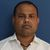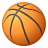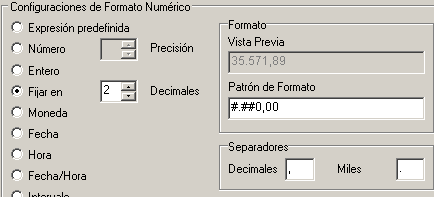# QlikView App Dev

Discussion Board for collaboration related to QlikView App Development.

Announcements
QlikWorld 2023, a live, in-person thrill ride. Save \$300 before February 6: REGISTER NOW!
cancel
Showing results for
Did you mean:Creator III

## how to use num() function in qlikview?

1 Solution

Accepted SolutionsPartner - Master II

# Num

num(expression [ , format-code [ , decimal-sep [ , thousands-sep ] ] ] )

The num function formats the expression numerically according to the string given as format-code. Decimal separator and thousands separator can be set as third and fourth parameters. If the parameters 2-4 are omitted, the number format set in the operating system is used.

Example:

The examples below assume the two following operating system settings:

 Default setting 1 Default setting 2 Number format # ##0,# #,##0.#

num( A, '0.0' ) where A=35648.375 returns:

 Setting 1 Setting 2 String 35 648 375 35648.375 Number 35648375 35648.375

num( A, '#,##0.##', '.' , ',' ) where A=35648 returns:

 Setting 1 Setting 2 String 35,648.00 35,648.00 Number 35648 35648

num( pi( ), '0,00' ) returns:

 Setting 1 Setting 2 String 3,14 003 Number 3.141592653 3.141592653
9 RepliesPartner - Master II

# Num

num(expression [ , format-code [ , decimal-sep [ , thousands-sep ] ] ] )

The num function formats the expression numerically according to the string given as format-code. Decimal separator and thousands separator can be set as third and fourth parameters. If the parameters 2-4 are omitted, the number format set in the operating system is used.

Example:

The examples below assume the two following operating system settings:

 Default setting 1 Default setting 2 Number format # ##0,# #,##0.#

num( A, '0.0' ) where A=35648.375 returns:

 Setting 1 Setting 2 String 35 648 375 35648.375 Number 35648375 35648.375

num( A, '#,##0.##', '.' , ',' ) where A=35648 returns:

 Setting 1 Setting 2 String 35,648.00 35,648.00 Number 35648 35648

num( pi( ), '0,00' ) returns:

 Setting 1 Setting 2 String 3,14 003 Number 3.141592653 3.141592653Partner - Master II

Note the difference : Num formats a number, Num# evaluates a text for example as number :

# Num#

num#(expression [ , format-code[ , decimal-sep [ , thousands-sep] ] ])

The num# function evaluates the expression numerically according to the string given as format-code. Decimal separator and thousands separator can be set as third and fourth parameters. If the parameters 2-4 are omitted, the default number format set by script variables or in the operating system is used.Not applicable

Is there a specific example you're looking to use the function to fix?MVP

It can be used to assign a specific format to your numeric field in a particular chart. For instance if you data within the chart is like this:

Dim 1=Sum(Value)
A12.362563253
B15.632634521

then you can use Num function to show only two decimal points like this:

Dim1=Num(Sum(Value), '#,##0.00')
A12.36
B15.63

Note: Num won't round the number for you, it will just truncate the number

So the basic idea is: Num helps in formatting a numeric field.

HTH

Best,

SMVP

Hi Sandip, Num is used to format numbers, it takes 2 parameters: number and format, ie:

Num(2000, '#.##0,00 €;-#.##0,00 €')

I think your best choice is go to the numbers tab of any chart and look how the numbers are formatted in the 'Format pattern' text boxCreator III
Author

What is the functionality of # and 0 here?MVP

Hi Sandip:

0 --> Means digit that has to be shown

# --> Digit is showed only if it's necesary

Examples to format the number 1:

Num(1, '00') will show 01 --> this format is useful to create dates, ie. Year(Today()) & Num(Month(Today()), '00')

Num(1, '##') will show 1Creator III
Author

can anyone give example of num# function? what is the difference between them?MVP

Hi, Num# is used to tell QV how to transform a string to a number.

In example in my country decimal separator is comma, so if i retrieve a number with dot as decimal separator QV can't understand the number and I have to use Num#() to tell the format.

If I use "=Num('10.2')+2" it returns null

If I use "=Num#('10.2', '0.0', '.', ',')+2" it returns "12,2"Community Browser